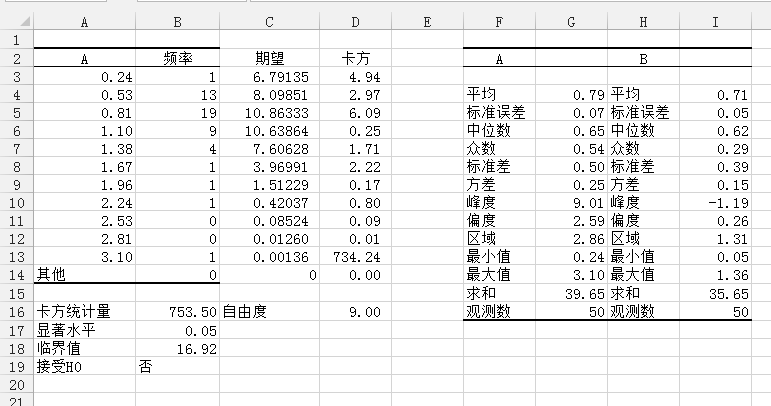# 实验数据的假设检验小记

#### 假设检验的基本知识

H0:$\mu=1$斤

#### 非参数假设检验

Example . 假设某次实验测得A,B两组50个数据如下, 试问他们是否可以认为他们是满足正态分布的?

A B
0.54 0.05
2.20 0.13
0.58 0.17
0.55 0.20
0.27 0.23
0.37 0.28
0.77 0.29
0.24 0.29
0.70 0.30
0.92 0.30
0.36 0.38
1.10 0.40
0.47 0.43
0.54 0.43
0.62 0.44
0.56 0.45
0.45 0.47
0.60 0.50
0.58 0.52
0.51 0.53
1.68 0.54
0.79 0.54
0.88 0.55
0.67 0.55
1.20 0.57
0.70 0.67
0.90 0.68
0.66 0.73
0.77 0.75
1.03 0.75
0.39 0.76
0.64 0.87
0.45 0.88
0.51 0.90
0.59 0.93
0.42 0.98
0.55 1.00
0.52 1.07
1.15 1.13
1.28 1.16
0.73 1.20
1.05 1.20
1.60 1.21
0.48 1.28
0.99 1.30
0.87 1.31
0.91 1.32
3.10 1.32
0.25 1.35
0.96 1.36

##### 直方图

###### 统计描述
1. 单击数据面板下的 数据分析， 选择描述统计
2. 输入区域内：选定待分析数据区域， 可以选择多行或者多列
3. 选择你将要分析的数据是以还是以的方式呈现的
4. 如果所选择的区域有数据标识， 勾选标识
5. 在输出区域选择输出到当前工作表还是新的工作表
6. 勾选汇总统计（输出基本统计量）
7. 确定便可生成统计描述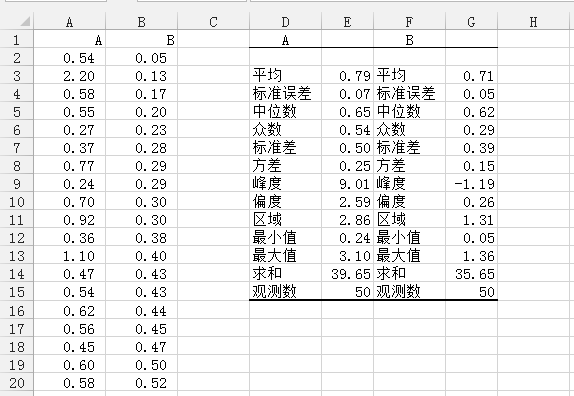###### 组数的计算

1. 方根: 数据个数的平方根并取整, Excel公式:=INT(SQRT(n)), 这里结果为7
2. Sturges 公式:数据个数关于$2$的对数取整加$1$, Excel公式:=INT(LOG(n,2))+1, 这里结果为6
3. Rice 准则: 数据个数的三次方根的二倍取整, Excel公式=INT(2*n^(1/3)), 这里结果为6
4. Doane 公式: 是Sturges公式的修正, 对处理异常数据有效. 计算公式为:
$$1 + \log_2( n ) + \log_2 \left( 1 + \frac { |g_1| }{\sigma_{g_1}} \right) ,$$
其中
$$\sigma_{g_1} = \sqrt { \frac { 6(n-2) }{ (n+1)(n+3) } }$$
而$g_1$为该分布的三阶矩偏度估计. 具体的计算可以在这个网页找到. 只需把你的数据(这里, 例如取第一列) 粘贴到网页的1 Copy&Paste your data下面的输入框, 然后单击 2. Please click here to start java applet 以加载java, 最后点击 3.Press Here to calculate the optimal bin size 我们就得到优化后的直方图. 在图上可以看到# of bins 10表示此时我们优化后的组数是10, 后面一个Bin width:0.286表示的是组距.

wiki上还有几种办法, 我这里直接跳过. 因为基本上利用第四种我们可以得到比较有效的组数.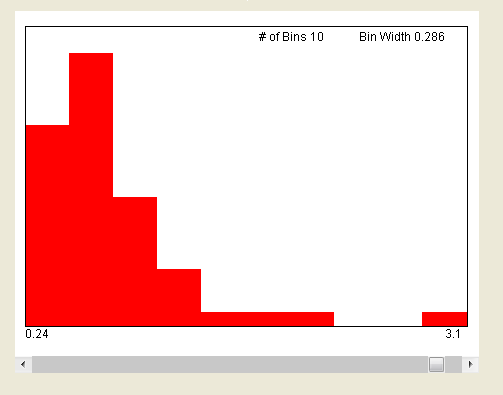###### 频数的统计

1. 参考第一个图, 在H1中输入:=E12, 并按F4切换到绝对引用, 这是因为A列的第一个分点就是最小值.
2. 接下来我们将用数据填充的办法计算后面的值, 于是只需设计好第二个公式, 在H2中中输入:=H2+$E$11/10, 意思很明显, 就是前一个数据(H2)加上组距, 这里组距等于区域(E11)除以组数10. 注意由于组数在下面填充中是不动的, 因此是绝对引用.
3. 填充直到一共有11个数为止(10个区间, 11个分点,呵呵~~), 这也可以通过最后一个值应该是最大值3.1(E13单元格给出)判断.
4. 至此, A列数据的仓库就做好了, 完全类似的做B列数据的. 注意这里组数是需要从新计算的, 这里利用组数计算的方法4结果有些奇葩, 竟然组数是2, 需要改进, 一般5-8比较正常, 这里选择6, 因为前面三种的计算有两种是6, 一种结果是7.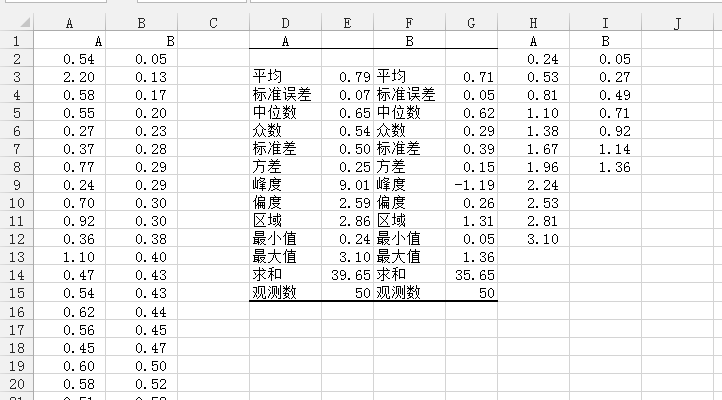###### 直方图的作法

1. 在J1中输入A的频数作为标题
2. 在J2中输入频数计算公式:=FREQUENCY(A2:A51,H2:H12)道理是很明显的, A2:A51表示待统计的数据, H2:H12表示仓库分组, 此时返回的是第一个仓库的频数.
3. 为了计算其他仓库的频数, 我们只需选中J2:J12, 再按F2, 最后按Ctrl+Shift+Enter即可得到其他仓库的频数.

1. 点击数据面板, 点击最后的数据分析, 在弹出的数据分析选框中, 选择直方图并确定.
2. 输入区域中选择A列数据$A$1:$A$51, 在接收区域中选择:$H$1:$H$12, 由于选择的数据中有表头, 我们需要勾选标志. 在输出选项中选择输出区域, 我们这里输出到当前工作表的$D$18. 最后勾选图表输出. 并确定
3. 请手动调整直方图的大小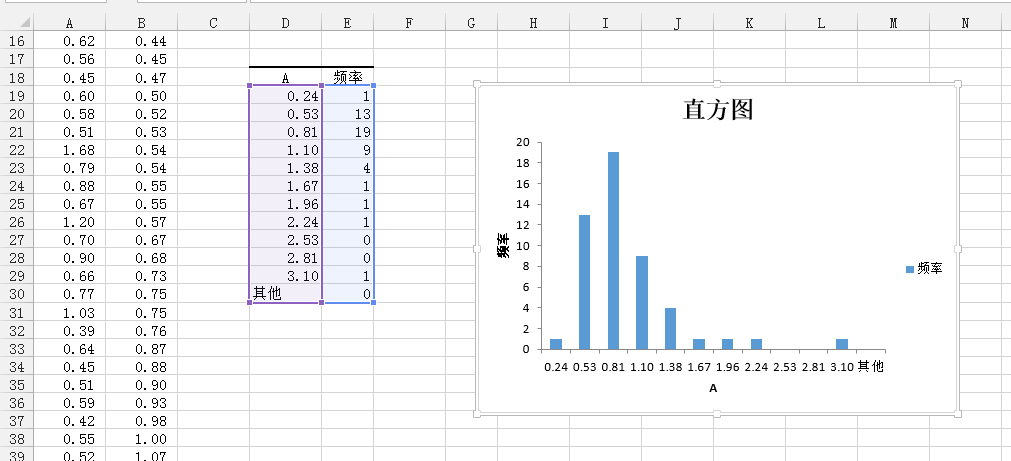##### 趋势线的添加

1. F18中输入正态图作为标题
2. F19中输入公式:=NORM.DIST(D19:D29,$E$3,$E$7,FALSE)计算第一个分点的正态分布之概率密度. 其中之所以选择D19:D29因为我们待会要用数组计算得到后面分点的概率密度. 而$E$3是A列数据的均值, $E$7是A列数据的标准差, 最后的FALSE表示我们计算的是概率密度而非累积密度.
总起来说, 就是计算A列数据作为正态分布在给定分点的概率密度是多少. 这样我们就可以做出A列数据的正态分布分布图了.
3. 计算其他分点的概率密度, 这可由前面讲过的数组计算公式得到: 选中F19:F29, 按下F2, 再按Ctrl+Shift+Enter即可

1. 在直方图的空白处单击以选中该直方图, 此时会看到设计面板, 单击以切换到该面板, 然后单击选择数据, 我们想要将正态图的数据添加到当前图表
2. 单击添加(A), 在系列名称里面输入=Sheet1!$F$18, 就是正态图的标题; 在系列值里选择=Sheet1!$F$19:$F$29(这里数据的添加都是点击后面带颜色的小框框, 然后再选择数据范围), 就是上面计算的正态分布的概率密度.
3. 两次确定, 即可在图表中看到添加进来的正态分布图, 但是此时比例不对(是按照实际数据的值来作图的, 即一个是频数最大为19, 而一个为概率密度, 最大为0.79), 这可以通过设置次坐标轴来调整.
4. 同样选中直方图(空白处单击), 切换到视图(事实上在图表空白处双击即可快速切换到视图面板), 单击更改图表类型, 在所有图表中选择组合, 可以看到频率系列默认的是簇状柱形图, 这个不用修改, 在正态图系列, 默认的是折线图, 我们将其修改为X Y(散点图)的第三个带平滑线的散点图, 并勾选后面的次坐标轴, 确定即可得到带正态分布的直方图.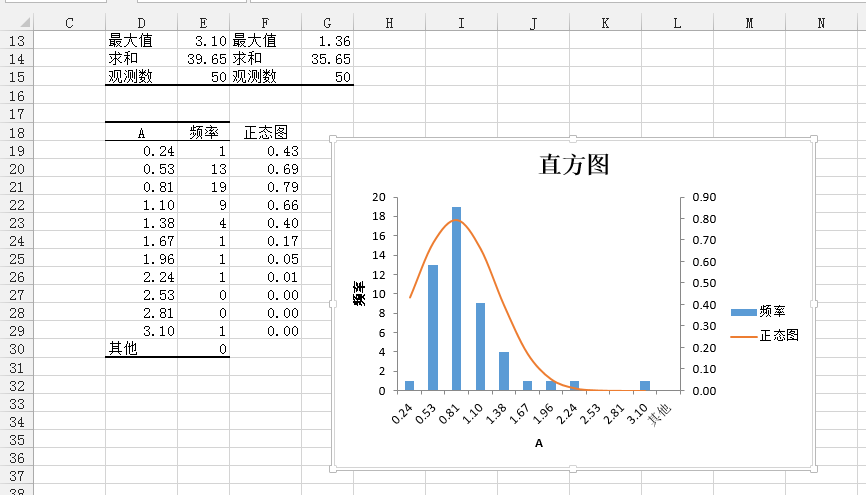##### $\chi^2$拟合检验

$\chi^2$拟合检验并不只是针对正态分布来检验的. 事实上, 观察上图(直方图的左边), 我们知道A列数据在每个仓库的频数, 例如: D19=0.24, E19=1, 表示的是A列数据中小于等于0.24的有1个, D20=0.53,E20=13表示大于0.24小于等于0.53的数据有13个, 与此类推, 最后的D30=其他, E30=1表示, 大于3.10的有1个.

$$\begin{equation} \chi^2=\sum_{i=1}^k\frac{(v_i-np_i)^2}{np_i}, \label{eq1} \end{equation}$$

1. 为了方便, 将A列数据的分组以及频数统计表(由直方图的作法自动生成, 位于直方图的左边)以及描述统计表复制到新的工作表(请参考最后的效果图)
2. 计算各个仓库的理论频数, 这需要特别处理第一个仓库和最后一个仓库(其他的哪一个), 首先来看第一个的理论频数计算公式, 在C3中输入:=$G$16*NORM.DIST(A3,$G$4,$G$8,TRUE), 其中G16就是数据总数, 它后面乘的是正态分布下第一个仓库的概率(还记得吗? 第一个仓库表示小于等于该值), 我想, 只需解释下最后一个参数(前面的参数已经说过), 这里的TRUE表示计算的是累积分布密度, 这是当然的, 因为该仓库表示的是小于等于0.24的数据.
3. 计算最后一个仓库的理论密度, 由于最后一个仓库表示的是大于该值的数据个数, 因此它对应的概率密度是1(密度之和为1哦)减去该数值的累积分布密度. 这样我们在C14中输入:=$G$16*(1-NORM.DIST($A$13,$G$4,$G$8,TRUE))
4. 计算中间仓库的理论频数, 在C4中输入:=$G$16*(NORM.DIST(A4,$G$4,$G$8,TRUE)-NORM.DIST(A3,$G$4,$G$8,TRUE)), 道理是很清楚的, 既然我们计算的是介于A3A4的频数, 当然就是样本容量(G16)乘以A4A3的累积分布密度之差.
5. 至此, 我们得到了理论频数(不一定是整数), 最后我们只需利用Excel提供的$\chi^2$概率的计算函数CHISQ.TEST来计算$\chi^2$检验的结果: 在A16输入公式:=CHISQ.TEST(B3:B14,C3:C14), 这里B3:B14是实际频数, C3:C14是理论频数, 这样Excel就会根据\eqref{eq1}来计算结果($\chi^2$分布的概率值$p$).

1. 统计推断(假设检验)的原假设$H_0$是对想要的证明的结论的否定, 那么这里$H_0$就是: 实验数据不服从正态分布.
2. 显著性水平是指当原假设(即$H_0$)实际上是正确的, 但根据统计推断, 人们却把它拒绝了的概率或风险.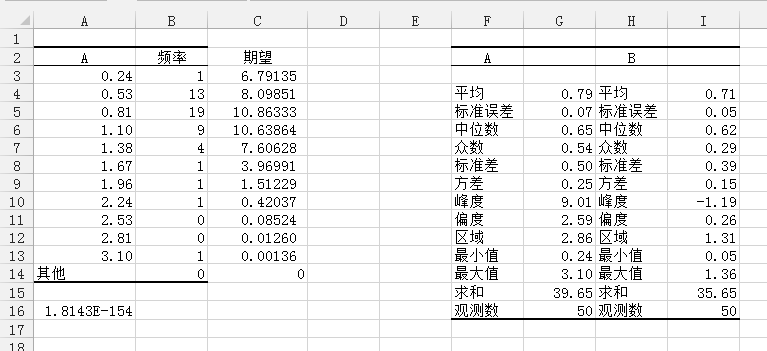##### 更正

1. D3中输入公式:=(B3-C3)^2/C3, 计算第一个仓库的频率与期望之差的平方除以期望
2. 向下填充到D14, 这就计算好了卡方统计量中的每一项
3. 求和, 在B16中求得卡方统计量的值:=SUM(D3:D14)
4. D16中求得自由度, =COUNT(B3:B14)-2-1
5. 在B17中设定显著水平, 例如我们想知道在显著水平为$0.05$时, 假设($H_0$)是否成立, 则在B17中输入0.05
6. B18中计算指定显著水平下的临界值, 计算公式为:=CHISQ.INV(1-B17,D16), 这里公式返回的是当$\chi^2$的累积概率密度达到$1-0.05$时的值, 后面的D16表示的是自由度, 因为这不是Excel默认的, 所有需要手动计算
7. 比较卡方统计量和临界值的大小, 因为理想情况下(即假设是正态分布时), 卡方统计量应该为$0$(期望频数和实际频数相等了), 所以如果卡方统计量小于临界值则接受原假设$H_0$(不是正态分布, 注意概率推断思想是从概率上反证, 因此我们原假设是:数据不服从正态分布, 即否定想要证明的命题: 数据是服从正态分布的), 否则拒绝原假设(是正态分布), 因此我们在B19中用一个IF来判断是否接受原假设:=IF(B16<B18,"是","否")